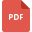# mathematical logic tutorialsPrime Numbers, LCM, HCFMental Maths Y2 Test 1
Mathematical Logic, India, Basic MathLinear and Nonlinear equations worksheet
Fundamental Math or Basic Math, Grade 8, India, Mathematical Analysis, Mathematical Logic, Linear Equationschange the subject of the formula worksheet
Mathematical Analysis, United States, Mathematical LogicRoyal-Russell-School-Sample-Year-7-Maths-Practice-Paper
Basic Math, Grade 7, United Kingdom, Applied Mathematics, Mathematical Analysis, Mathematical LogicIGCSE Math 2019
Mathematical Logic, England, Mathematical Analysis, Fundamental Math or Basic Math